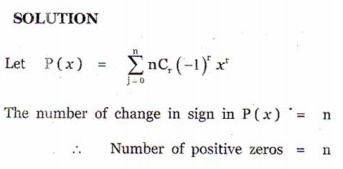# Chapter 3: Theory of Equations - Online Test

Q1. A zero of x3 + 64 is
Explaination / Solution:Q2. If f and g are polynomials of degrees m and n respectively, and if h(x) = ( f o g )(x) , then the degree of h is
Explaination / Solution: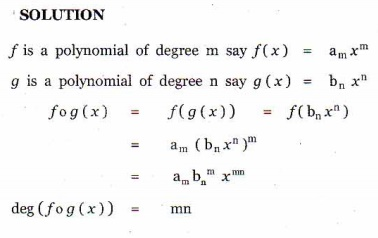Q3. A polynomial equation in x of degree n always has
Explaination / Solution:

A polynomial of degree n always has n roots
Q4. If α , β , and  γ  are the zeros of  x+ px+ qx + r , then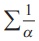is
Explaination / Solution: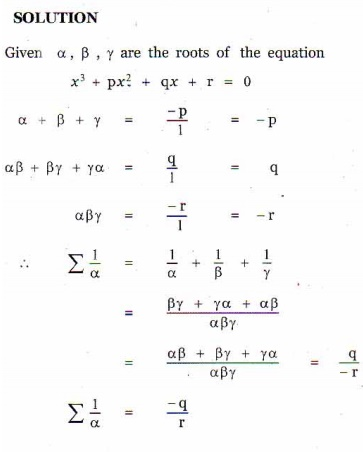Q5. According to the rational root theorem, which number is not possible rational zero of 4x+ 2x4 10x3 5 ?
Explaination / Solution:Q6. The polynomial x3 kx2 + 9x has three real zeros if and only if, k satisfies
Explaination / Solution: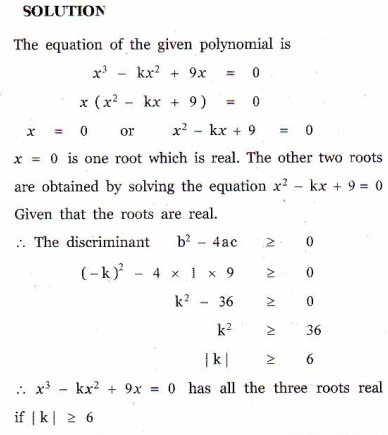Q7. The number of real numbers in [0, 2π] satisfying sin4 x 2 sin2 x +1 is
Explaination / Solution:Q8. If x3 +12x2 +10ax +1999 definitely has a positive zero, if and only if
Explaination / Solution:Q9. The polynomial x3 + 2x + 3 has
Explaination / Solution:Q10. The number of positive zeros of the polynomial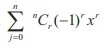is
Explaination / Solution: